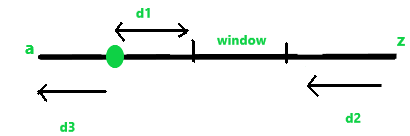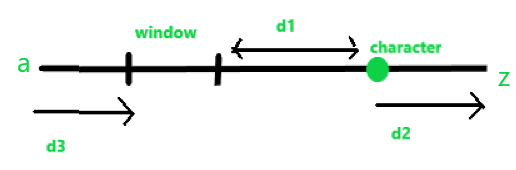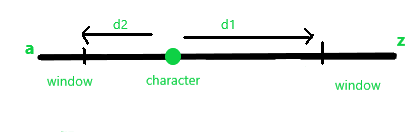# Minimum cost to modify a string

• Difficulty Level : Hard
• Last Updated : 27 May, 2021

Given string str consisting of lower case alphabets only and an integer K. The task is to find the minimum cost to modify the string such that the ASCII value difference between any two characters of the given string is less than equal to K
The following operations can be performed on the string:

1. Increasing a character: For example, when you increase ‘a’ by 1 it becomes ‘b’. Similarly, if you increase ‘z’ by 1 it becomes ‘a’. The increment is done in a cyclic manner.
2. Decreasing a character: For example, when you decrease ‘a’ by 1 it becomes ‘z’. Similarly, if you decrease ‘z’ by 1 it becomes ‘y’. Decrement is done in a cyclic manner.

Examples:

Input: str = “abcd”, K = 1
Output:
Change ‘a’ to ‘b’ with cost 1 and ‘d’ to ‘c’ again at cost 1.
Total cost = 1 + 1 = 2.
The modified string will be “bbcc”

Input: str = “abcdefghi”, K = 2
Output: 12

Approach: The idea is to maintain a hash table for all the characters of the string to reduce time complexity instead of taking one character at a time. We need to check all the windows containing k contiguous characters and find the minimum cost among all the windows for modifying all the characters of the string.
The cost of modification of a character falls under the following categories:

• Case 1: If the characters are within the window, they will not incur any cost for modifying them.
• Case 2: If the window is in between the characters a-z and the character is on the left side of the window.• If we increase the character which will incur a cost of d1 or if we decrease the character which will incur a cost of d2+d3, we find a minimum of d1 and d2+d3.
If the window is in between the characters a-z and the character is on the right side of the window.• If we decrease the character which will incur a cost of d1 or if we increase the character which will incur a cost of d2+d3, we find a minimum of d1 and d2+d3.

• Case 3: If the window is a sub-window of characters ending at z and starting from a and if the character is outside the window.• If we increase the character, it will incur a cost of d1 and if we decrease the character it will incur a cost of d2, we find a minimum between d1 and d2.

Below is the implementation of the above approach:

## C++

 `// C++ implementation of the approach` `#include ` `using` `namespace` `std;`   `// Function to return the minimum cost` `int` `minCost(string str, ``int` `K)` `{` `    ``int` `n = str.length();`   `    ``// Initialize result` `    ``int` `res = 999999999, count = 0, a, b;`   `    ``// To store the frequency of characters` `    ``// of the string` `    ``int` `cnt;`   `    ``// Initialize array with 0` `    ``memset``(cnt, 0, ``sizeof``(cnt));`   `    ``// Update the frequencies of the` `    ``// characters of the string` `    ``for` `(``int` `i = 0; i < n; i++)` `        ``cnt[str[i] - ``'a'` `+ 1]++;`   `    ``// Loop to check all windows from a-z` `    ``// where window size is K` `    ``for` `(``int` `i = 1; i < (26 - K + 1); i++) {`   `        ``// Starting index of window` `        ``a = i;`   `        ``// Ending index of window` `        ``b = i + K;` `        ``count = 0;` `        ``for` `(``int` `j = 1; j <= 26; j++) {`   `            ``// Check if the string contains character` `            ``if` `(cnt[j] > 0) {`   `                ``// Check if the character is on left side of window` `                ``// find the cost of modification for character` `                ``// add value to count` `                ``// calculate nearest distance of modification` `                ``if` `(j >= a && j >= b)` `                    ``count = count + (min(j - b, 25 - j + a + 1)) * cnt[j];`   `                ``// Check if the character is on right side of window` `                ``// find the cost of modification for character` `                ``// add value to count` `                ``// calculate nearest distance of modification` `                ``else` `if` `(j <= a && j <= b)` `                    ``count = count + (min(a - j, 25 + j - b + 1)) * cnt[j];` `            ``}` `        ``}`   `        ``// Find the minimum of all costs` `        ``// for modifying the string` `        ``res = min(res, count);` `    ``}`   `    ``// Loop to check all windows` `    ``// Here window contains characters` `    ``// before z and after z of window size K` `    ``for` `(``int` `i = 26 - K + 1; i <= 26; i++) {`   `        ``// Starting index of window` `        ``a = i;`   `        ``// Ending index of window` `        ``b = (i + K) % 26;` `        ``count = 0;`   `        ``for` `(``int` `j = 1; j <= 26; j++) {`   `            ``// Check if the string contains character` `            ``if` `(cnt[j] > 0) {`   `                ``// If characters are outside window` `                ``// find the cost for modifying character` `                ``// add value to count` `                ``if` `(j >= b and j <= a)` `                    ``count = count + (min(j - b, a - j)) * cnt[j];` `            ``}` `        ``}`   `        ``// Find the minimum of all costs` `        ``// for modifying the string` `        ``res = min(res, count);` `    ``}`   `    ``return` `res;` `}`   `// Driver code` `int` `main()` `{` `    ``string str = ``"abcdefghi"``;` `    ``int` `K = 2;` `    ``cout << minCost(str, K);`   `    ``return` `0;` `}`

## Java

 `// Java program to implement` `// the above approach` `import` `java.util.Arrays;`   `class` `GFG` `{`   `// Function to return the minimum cost` `static` `int` `minCost(String str, ``int` `K)` `{` `    ``int` `n = str.length();`   `    ``// Initialize result` `    ``int` `res = ``999999999``, count = ``0``, a, b;`   `    ``// To store the frequency of characters` `    ``// of the string` `    ``int` `cnt[] = ``new` `int``[``27``];`   `    ``// Initialize array with 0` `    ``Arrays.fill(cnt, ``0``);`   `    ``// Update the frequencies of the` `    ``// characters of the string` `    ``for` `(``int` `i = ``0``; i < n; i++)` `        ``cnt[str.charAt(i) - ``'a'` `+ ``1``]++;`   `    ``// Loop to check all windows from a-z` `    ``// where window size is K` `    ``for` `(``int` `i = ``1``; i < (``26` `- K + ``1``); i++) ` `    ``{`   `        ``// Starting index of window` `        ``a = i;`   `        ``// Ending index of window` `        ``b = i + K;` `        ``count = ``0``;` `        ``for` `(``int` `j = ``1``; j <= ``26``; j++) ` `        ``{`   `            ``// Check if the string contains character` `            ``if` `(cnt[j] > ``0``)` `            ``{`   `                ``// Check if the character is on left side of window` `                ``// find the cost of modification for character` `                ``// add value to count` `                ``// calculate nearest distance of modification` `                ``if` `(j >= a && j >= b)` `                    ``count = count + (Math.min(j - b, ` `                            ``25` `- j + a + ``1``)) * cnt[j];`   `                ``// Check if the character is on right side of window` `                ``// find the cost of modification for character` `                ``// add value to count` `                ``// calculate nearest distance of modification` `                ``else` `if` `(j <= a && j <= b)` `                    ``count = count + (Math.min(a - j,` `                            ``25` `+ j - b + ``1``)) * cnt[j];` `            ``}` `        ``}`   `        ``// Find the minimum of all costs` `        ``// for modifying the string` `        ``res = Math.min(res, count);` `    ``}`   `    ``// Loop to check all windows` `    ``// Here window contains characters` `    ``// before z and after z of window size K` `    ``for` `(``int` `i = ``26` `- K + ``1``; i <= ``26``; i++)` `    ``{`   `        ``// Starting index of window` `        ``a = i;`   `        ``// Ending index of window` `        ``b = (i + K) % ``26``;` `        ``count = ``0``;`   `        ``for` `(``int` `j = ``1``; j <= ``26``; j++)` `        ``{`   `            ``// Check if the string contains character` `            ``if` `(cnt[j] > ``0``)` `            ``{`   `                ``// If characters are outside window` `                ``// find the cost for modifying character` `                ``// add value to count` `                ``if` `(j >= b && j <= a)` `                    ``count = count + (Math.min(j - b, a - j)) * cnt[j];` `            ``}` `        ``}`   `        ``// Find the minimum of all costs` `        ``// for modifying the string` `        ``res = Math.min(res, count);` `    ``}`   `    ``return` `res;` `}`   `// Driver code` `public` `static` `void` `main(String[] args)` `{` `    ``String str = ``"abcdefghi"``;` `    ``int` `K = ``2``;` `    ``System.out.println(minCost(str, K));` `}` `}`   `/* This code contributed by PrinciRaj1992 */`

## Python3

 `# Python 3 implementation of the approach`   `# Function to return the minimum cost` `def` `minCost(str1, K):` `    ``n ``=` `len``(str1)`   `    ``# Initialize result` `    ``res ``=` `999999999` `    ``count ``=` `0`   `    ``# To store the frequency of characters` `    ``# of the string` `    ``cnt ``=` `[``0` `for` `i ``in` `range``(``27``)]`   `    ``# Update the frequencies of the` `    ``# characters of the string` `    ``for` `i ``in` `range``(n):` `        ``cnt[``ord``(str1[i]) ``-` `ord``(``'a'``) ``+` `1``] ``+``=` `1`   `    ``# Loop to check all windows from a-z` `    ``# where window size is K` `    ``for` `i ``in` `range``(``1``, ``26` `-` `K ``+` `1``, ``1``):` `        ``# Starting index of window` `        ``a ``=` `i`   `        ``# Ending index of window` `        ``b ``=` `i ``+` `K` `        ``count ``=` `0` `        ``for` `j ``in` `range``(``1``, ``27``, ``1``):` `            `  `            ``# Check if the string contains character` `            ``if` `(cnt[j] > ``0``):` `                `  `                ``# Check if the character is on left side of window` `                ``# find the cost of modification for character` `                ``# add value to count` `                ``# calculate nearest distance of modification` `                ``if` `(j >``=` `a ``and` `j >``=` `b):` `                    ``count ``=` `count ``+` `(``min``(j ``-` `b, ``25` `-` `                                         ``j ``+` `a ``+` `1``)) ``*` `cnt[j]`   `                ``# Check if the character is on right side of window` `                ``# find the cost of modification for character` `                ``# add value to count` `                ``# calculate nearest distance of modification` `                ``elif` `(j <``=` `a ``and` `j <``=` `b):` `                    ``count ``=` `count ``+` `(``min``(a ``-` `j, ``25` `+` `                                             ``j ``-` `b ``+` `1``)) ``*` `cnt[j]`   `        ``# Find the minimum of all costs` `        ``# for modifying the string` `        ``res ``=` `min``(res, count)`   `    ``# Loop to check all windows` `    ``# Here window contains characters` `    ``# before z and after z of window size K` `    ``for` `i ``in` `range``(``26` `-` `K ``+` `1``, ``27``, ``1``):` `        `  `        ``# Starting index of window` `        ``a ``=` `i`   `        ``# Ending index of window` `        ``b ``=` `(i ``+` `K) ``%` `26` `        ``count ``=` `0`   `        ``for` `j ``in` `range``(``1``, ``27``, ``1``):` `            `  `            ``# Check if the string contains character` `            ``if` `(cnt[j] > ``0``):` `                `  `                ``# If characters are outside window` `                ``# find the cost for modifying character` `                ``# add value to count` `                ``if` `(j >``=` `b ``and` `j <``=` `a):` `                    ``count ``=` `count ``+` `(``min``(j ``-` `b, ` `                                         ``a ``-` `j)) ``*` `cnt[j]`   `        ``# Find the minimum of all costs` `        ``# for modifying the string` `        ``res ``=` `min``(res, count)`   `    ``return` `res`   `# Driver code` `if` `__name__ ``=``=` `'__main__'``:` `    ``str1 ``=` `"abcdefghi"` `    ``K ``=` `2` `    ``print``(minCost(str1, K))`   `# This code is contributed by` `# Surendra_Gangwar`

## C#

 `// C# program to implement` `// the above approach` `using` `System;`   `class` `GFG` `{`   `// Function to return the minimum cost` `static` `int` `minCost(String str, ``int` `K)` `{` `    ``int` `n = str.Length;`   `    ``// Initialize result` `    ``int` `res = 999999999, count = 0, a, b;`   `    ``// To store the frequency of characters` `    ``// of the string` `    ``int` `[]cnt = ``new` `int``;`     `    ``// Update the frequencies of the` `    ``// characters of the string` `    ``for` `(``int` `i = 0; i < n; i++)` `        ``cnt[str[i] - ``'a'` `+ 1]++;`   `    ``// Loop to check all windows from a-z` `    ``// where window size is K` `    ``for` `(``int` `i = 1; i < (26 - K + 1); i++) ` `    ``{`   `        ``// Starting index of window` `        ``a = i;`   `        ``// Ending index of window` `        ``b = i + K;` `        ``count = 0;` `        ``for` `(``int` `j = 1; j <= 26; j++) ` `        ``{`   `            ``// Check if the string contains character` `            ``if` `(cnt[j] > 0)` `            ``{`   `                ``// Check if the character is on left side of window` `                ``// find the cost of modification for character` `                ``// add value to count` `                ``// calculate nearest distance of modification` `                ``if` `(j >= a && j >= b)` `                    ``count = count + (Math.Min(j - b, ` `                            ``25 - j + a + 1)) * cnt[j];`   `                ``// Check if the character is on right side of window` `                ``// find the cost of modification for character` `                ``// add value to count` `                ``// calculate nearest distance of modification` `                ``else` `if` `(j <= a && j <= b)` `                    ``count = count + (Math.Min(a - j,` `                            ``25 + j - b + 1)) * cnt[j];` `            ``}` `        ``}`   `        ``// Find the minimum of all costs` `        ``// for modifying the string` `        ``res = Math.Min(res, count);` `    ``}`   `    ``// Loop to check all windows` `    ``// Here window contains characters` `    ``// before z and after z of window size K` `    ``for` `(``int` `i = 26 - K + 1; i <= 26; i++)` `    ``{`   `        ``// Starting index of window` `        ``a = i;`   `        ``// Ending index of window` `        ``b = (i + K) % 26;` `        ``count = 0;`   `        ``for` `(``int` `j = 1; j <= 26; j++)` `        ``{`   `            ``// Check if the string contains character` `            ``if` `(cnt[j] > 0)` `            ``{`   `                ``// If characters are outside window` `                ``// find the cost for modifying character` `                ``// add value to count` `                ``if` `(j >= b && j <= a)` `                    ``count = count + (Math.Min(j - b, a - j)) * cnt[j];` `            ``}` `        ``}`   `        ``// Find the minimum of all costs` `        ``// for modifying the string` `        ``res = Math.Min(res, count);` `    ``}`   `    ``return` `res;` `}`   `// Driver code` `public` `static` `void` `Main(String[] args)` `{` `    ``String str = ``"abcdefghi"``;` `    ``int` `K = 2;` `    ``Console.WriteLine(minCost(str, K));` `}` `}`   `// This code has been contributed by 29AjayKumar`

## PHP

 ` 0)` `            ``{ `   `                ``// Check if the character is on left side of window ` `                ``// find the cost of modification for character ` `                ``// add value to count ` `                ``// calculate nearest distance of modification ` `                ``if` `(``\$j` `>= ``\$a` `&& ``\$j` `>= ``\$b``) ` `                    ``\$count` `= ``\$count` `+ (min(``\$j` `- ``\$b``, 25 - ``\$j` `+ ``\$a` `+ 1)) * ``\$cnt``[``\$j``]; `   `                ``// Check if the character is on right side of window ` `                ``// find the cost of modification for character ` `                ``// add value to count ` `                ``// calculate nearest distance of modification ` `                ``else` `if` `(``\$j` `<= ``\$a` `&& ``\$j` `<=``\$b``) ` `                    ``\$count` `= ``\$count` `+ (min(``\$a` `- ``\$j``, 25 + ``\$j` `- ``\$b` `+ 1)) * ``\$cnt``[``\$j``]; ` `            ``} ` `        ``} `   `        ``// Find the minimum of all costs ` `        ``// for modifying the string ` `        ``\$res` `= min(``\$res``, ``\$count``); ` `    ``} `   `    ``// Loop to check all windows ` `    ``// Here window contains characters ` `    ``// before z and after z of window size K ` `    ``for` `(``\$i` `= 26 - ``\$K` `+ 1; ``\$i` `<= 26; ``\$i``++) ` `    ``{ `   `        ``// Starting index of window ` `        ``\$a` `= ``\$i``; `   `        ``// Ending index of window ` `        ``\$b` `= (``\$i` `+ ``\$K``) % 26; ` `        ``\$count` `= 0; `   `        ``for` `(``\$j` `= 1; ``\$j` `<= 26; ``\$j``++) ` `        ``{ `   `            ``// Check if the string contains character ` `            ``if` `(``\$cnt``[``\$j``] > 0)` `            ``{ `   `                ``// If characters are outside window ` `                ``// find the cost for modifying character ` `                ``// add value to count ` `                ``if` `(``\$j` `>= ``\$b` `and` `\$j` `<= ``\$a``) ` `                    ``\$count` `= ``\$count` `+ (min(``\$j` `- ``\$b``,``\$a` `- ``\$j``)) * ``\$cnt``[``\$j``]; ` `            ``} ` `        ``} `   `        ``// Find the minimum of all costs ` `        ``// for modifying the string ` `        ``\$res` `= min(``\$res``, ``\$count``); ` `    ``} `   `    ``return` `\$res``; ` `} `   `    ``// Driver code ` `    ``\$str` `= ``"abcdefghi"``; ` `    ``\$K` `= 2; ` `    ``echo` `minCost(``\$str``, ``\$K``); ` `    `  `    ``// This code is contributed by Ryuga`   `?>`

## Javascript

 ``

Output:

`12`

Time Complexity: O(n)
Auxiliary Space: O(n)

My Personal Notes arrow_drop_up
Recommended Articles
Page :